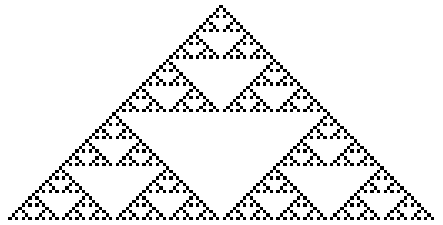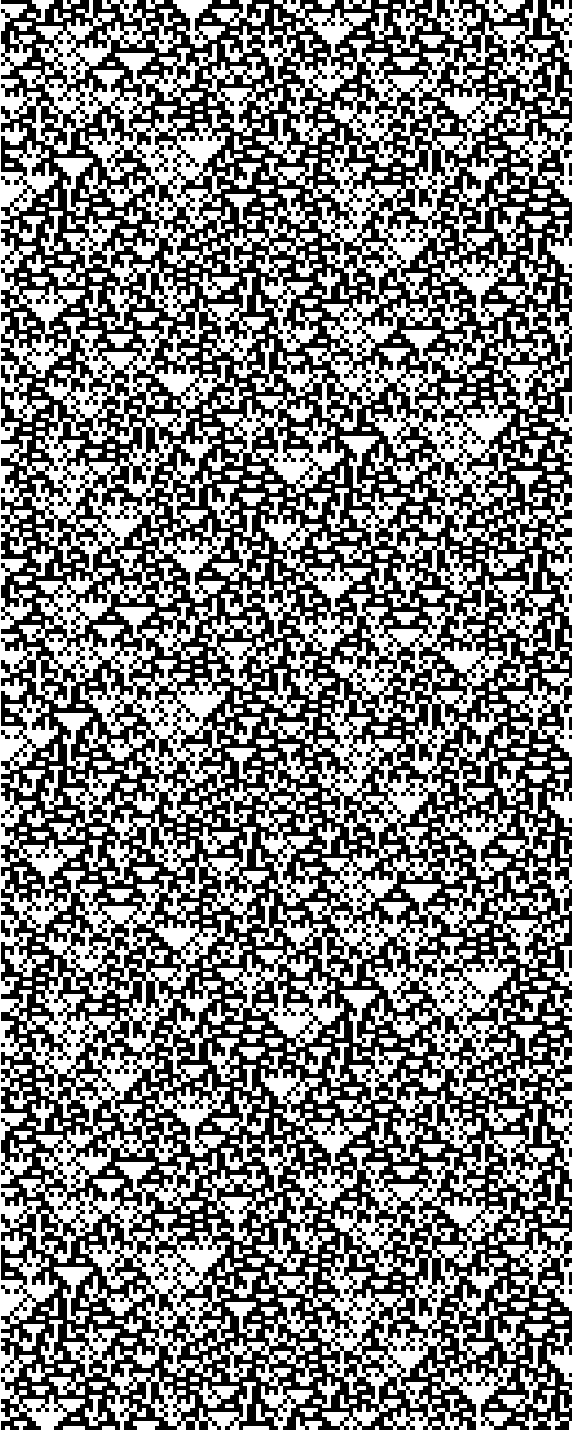# Sierpinski triangle strikes again

A couple months ago I wrote about how a simple random process gives rise to the Sierpinski triangle. Draw an equilateral triangle and pick a random point in the plane. Repeatedly pick a triangle vertex at random and move half way from the current position to that vertex. The result converges to a Sierpinksi triangle. This post will show another way to arrive at the same pattern using cellular automata.

Imagine an infinite grid of graph paper and fill in a few squares in one row. The squares in the subsequent rows are filled in or not depending on the state of the square’s upstairs neighbors. For elementary cellular automata, the state of a square depends on the square directly above and the two squares diagonally above on each side. In matrix notation, the state of the (ij) element depends on elements (i-1, j-1), (i-1, j), and (i-1, j+1).

There are 256 possible elementary cellular automata, and here’s how you can number them. The states of three consecutive cells determine a three-bit binary number. An automaton is determined by what bit it assigned to each of the eight possible three-bit states, so an automaton corresponds to an 8-bit number. In this post we’re interested in Rule 90. In binary, 90 is written 01011010, and the table below spells out the rule in detail.

```000 -> 0
001 -> 1
010 -> 0
011 -> 1
100 -> 1
101 -> 0
110 -> 1
111 -> 0
```

If we start with a single square filled in (i.e. set to 1) then this is the graph we get, i.e. the Sierpenski triangle:This pattern depends critically on our initial conditions. Roughly speaking, it seems that if you start with regular initial conditions you’ll get regular results. If you start with random initial conditions, you’ll get random-looking results as shown below.We see the same empty triangles as before, but they’re much smaller and appear scattered throughout.

In order to create a rectangular image, I wrapped the edges: the upper left neighbor of a point on the left edge is the right-most square on the row above, and similarly for the right edge. You could think of this as wrapping our graph paper into a cylinder.

## 3 thoughts on “Sierpinski triangle strikes again”

1. Thanks. I don’t think I’ve seen that.

2. Rule 90 can be summarized as adding the first and last bits (mod 2). This is the exact same rule as Pascal’s triangle, which is why you also see the same pattern taking Pascal’s triangle modulo 2.